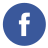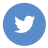### Java Basic Operators

java

In Java, operators are symbols that are used to perform operations on variables and values. There are several types of operators in Java, including:

1. Arithmetic operators: These operators are used to perform arithmetic operations such as addition, subtraction, multiplication, and division.

`+`: addition
`-`: subtraction
`*`: multiplication
`/`: division
`%`: remainder (modulus)

1. Comparison operators: These operators are used to compare two values and return a boolean result.

`==`: equal to
`!=`: not equal to
`&gt;` : greater than
`&lt;`: less than
`&gt;=`: greater than or equal to
`&lt;=`: less than or equal to

1. Logical operators: These operators are used to perform logical operations such as AND, OR, and NOT.

`&&`: AND
`||`: OR
`!`: NOT

1. Assignment operators: These operators are used to assign a value to a variable.

`=`: assignment
`+=`: addition assignment
`-=`: subtraction assignment
`*=`: multiplication assignment
`/=`: division assignment
`%=`: remainder assignment

1. Increment and decrement operators: These operators are used to increment or decrement a value by 1.

`++`: increment
`--`: decrement

Here is an example of how to use some of these operators in Java:

``````int x = 10;
int y = 20;
int z = x + y; // z is 30
int a = x - y; // a is -10
int b = x * y; // b is 200
int c = y / x; // c is 2
int d = y % x; // d is 0

boolean e = x == y; // e is false
boolean f = x != y; // f is true
boolean g = x > y; // g is false
boolean h = x < y; // h is true

x += y; // x is 30
y -= x; // y is -10
z *= x; // z is 900
a /= y; // a is -2
b %= d; // b is 0

x++; // x is 31
y--; // y is -11``````